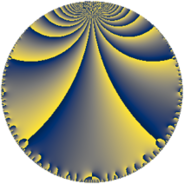# Properties

 Label 23.6.cLevel $23$ Weight $6$ Character orbit 23.c Rep. character $\chi_{23}(2,\cdot)$ Character field $\Q(\zeta_{11})$ Dimension $90$ Newform subspaces $1$ Sturm bound $12$ Trace bound $0$

# Related objects

## Defining parameters

 Level: $$N$$ $$=$$ $$23$$ Weight: $$k$$ $$=$$ $$6$$ Character orbit: $$[\chi]$$ $$=$$ 23.c (of order $$11$$ and degree $$10$$) Character conductor: $$\operatorname{cond}(\chi)$$ $$=$$ $$23$$ Character field: $$\Q(\zeta_{11})$$ Newform subspaces: $$1$$ Sturm bound: $$12$$ Trace bound: $$0$$

## Dimensions

The following table gives the dimensions of various subspaces of $$M_{6}(23, [\chi])$$.

Total New Old
Modular forms 110 110 0
Cusp forms 90 90 0
Eisenstein series 20 20 0

## Trace form

 $$90 q - 11 q^{2} - 7 q^{3} - 139 q^{4} + 5 q^{5} - 148 q^{6} - 29 q^{7} - 44 q^{8} - 894 q^{9} + O(q^{10})$$ $$90 q - 11 q^{2} - 7 q^{3} - 139 q^{4} + 5 q^{5} - 148 q^{6} - 29 q^{7} - 44 q^{8} - 894 q^{9} + 155 q^{10} - 89 q^{11} + 276 q^{12} + 313 q^{13} + 957 q^{14} - 4232 q^{15} + 5637 q^{16} + 5256 q^{17} + 11184 q^{18} - 3622 q^{19} - 2135 q^{20} - 6000 q^{21} - 10050 q^{22} - 11740 q^{23} - 20042 q^{24} - 3312 q^{25} + 432 q^{26} + 18236 q^{27} + 42219 q^{28} + 820 q^{29} + 39879 q^{30} - 10618 q^{31} - 44595 q^{32} - 52342 q^{33} - 48583 q^{34} - 12737 q^{35} + 62095 q^{36} + 37741 q^{37} + 93044 q^{38} + 51241 q^{39} + 54519 q^{40} + 27731 q^{41} - 74509 q^{42} - 24555 q^{43} - 93314 q^{44} - 97906 q^{45} - 178395 q^{46} - 72522 q^{47} - 84106 q^{48} - 60450 q^{49} + 100394 q^{50} + 92167 q^{51} + 289884 q^{52} + 37321 q^{53} + 74406 q^{54} + 24327 q^{55} + 73828 q^{56} + 210858 q^{57} - 46295 q^{58} + 12675 q^{59} + 40595 q^{60} + 169431 q^{61} + 126424 q^{62} + 48782 q^{63} - 152118 q^{64} - 204392 q^{65} - 289970 q^{66} - 68547 q^{67} - 619298 q^{68} - 269580 q^{69} - 616314 q^{70} - 116130 q^{71} - 90563 q^{72} - 41005 q^{73} - 39958 q^{74} - 89428 q^{75} - 54510 q^{76} + 473172 q^{77} + 749704 q^{78} + 562545 q^{79} + 1784628 q^{80} + 1038036 q^{81} + 361674 q^{82} + 235154 q^{83} + 314877 q^{84} - 216047 q^{85} - 896543 q^{86} - 516271 q^{87} - 213359 q^{88} - 497007 q^{89} - 1378102 q^{90} - 630132 q^{91} - 861506 q^{92} - 1401408 q^{93} - 497781 q^{94} - 605790 q^{95} - 1213923 q^{96} - 292308 q^{97} + 943825 q^{98} + 525394 q^{99} + O(q^{100})$$

## Decomposition of $$S_{6}^{\mathrm{new}}(23, [\chi])$$ into newform subspaces

Label Dim $A$ Field CM Traces $q$-expansion
$a_{2}$ $a_{3}$ $a_{5}$ $a_{7}$
23.6.c.a $90$ $3.689$ None $$-11$$ $$-7$$ $$5$$ $$-29$$